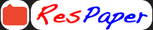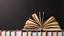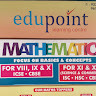Trending ▼   ResFinder# ISC Class XII Prelims 2020 : Mathematics (Gokuldham High School & Junior College (GHS), Mumbai)

5 pages, 52 questions, 23 questions with responses, 24 total responses,20Abyl +Fave Message ProfileTimelineUploads
 Home > alo2 >   F Also featured on: School Page isc and 1 moreFormatting page ...

GOKULDHAM HIGH SCHOOL & JUNIOR COLLEGE MATHEMATICS [ II PRELIMS 13-01-2020 ] (Maximum Marks : 100) (Time allowed : Three hours) (Candidates are allowed additional 15 minutes for only reading the paper. They must NOT start writing during this time.) ----------------------------------------------------------------------------------------------The Question Paper consists of three sections A, B and C Candidates are required to attempt all the questions from Section A and all the questions EITHER from Section B OR Section C. Section A : Internal choice has been provided in three questions of four mark each and two questions of six marks each. Section B : Internal choice has been provided in two questions of four mark each. Section C : Internal choice has been provided in two questions of four mark each. All working, including rough work, should be done on the same sheet as, and adjacent to, the rest of the answer. The intended marks for questions or parts of questions are given in brackets [ ]. Mathematical tables and squared paper are provided. Slide rule may be used. -----------------------------------------------------------------------------------------------SECTION A [80 Marks] Question 1 [10x2] i) If the binary operation * on the set of R of real numbers defined by 3 a * b = , write the identity element in R for * 7 ii) Solve cos 1 ( cos 1 ) = iii) For what value of x the following matrix is singular? 5 +1 [ ] 2 4 Using properties of determinants, solve for x + | + | + Find the differential equation of the family of curves y = A + B , where A and B are arbitrary constants. Using L hospital s rule , Evaluate :lim [ ] iv) v) vi) 6 vii) viii) ix) x) 2 2 Evaluate : 02 log dx Given that two numbers appearing on throwing two dice are different. find the probability of the event the sum of the numbers on the dice is 4. Given that the events E and F are such that P(E) = P (E F) and P(F) = p Find p if E and F are independent events. Write the equation of the normal to the curve y = 2 2 + 3 Sin x at x= 0 ---------------------------------------------------------------------------------------------------This paper consists of 5 printed pages. 1220 860 ISC XII

Related ResPapers
 ISC Class XII MID-TERM 2022 : Mathematics (Bombay Scottish School, Mahim, Mumbai) by iqbalmohd ISC Class XII Prelims 2020 : Mathematics (Hill Top School, Jamshedpur) by sanjay_102 ISC Class XII Prelims 2021 : Mathematics (St. Teresas Secondary School (STSS), Kidderpore, Kolkata) by liyajacob ISC Class XII Prelims 2020 : Mathematics (Brightlands School, Dehradun) by dyfiFormatting page ...

Top Contributors
to this ResPaperRishikesh Balaji(21)Liya Jacob(2)Sree Devi(1)ResPaper Admins(1)Formatting page ...Formatting page ...Formatting page ...